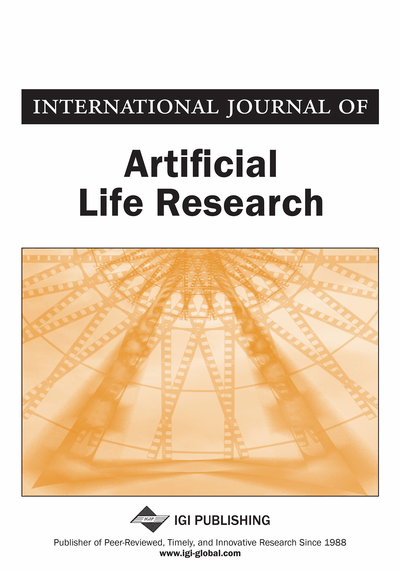# Modeling Fractal Structure of Systems of Cities Using Spatial Correlation Function

Yanguang Chen (Peking University, China) and Shiguo Jiang (The Ohio State University, USA)
DOI: 10.4018/jalr.2010102102
Available
\$37.50
No Current Special Offers

## Abstract

This article proposes a new method to analyze the spatial structure of urban systems using ideas from fractals. Regarding a system of cities as a set of “particles” distributed randomly on a triangular lattice, we construct a spatial correlation function of cities. Suppose that the spatial correlation follows the power law. It can be proved that the correlation exponent is the second order generalized dimension. The spatial correlation model is applied to the system of cities in China. The results show that the Chinese urban system can be described by the correlation dimension ranging from 1.3 to 1.6. The fractality of self-organized network of cities in both the conventional geographic space and the “time” space is revealed with the empirical evidence. The spatial correlation analysis is significant in that it is applicable to both large and small sizes of samples and can be used to link different fractal dimensions in urban study, including box dimension and radial dimension.
Article Preview
Top

## Introduction

The evolution of cities as systems and systems of cities bears some similarity. In theory, a system of cites follows the same spatial scaling laws with a city as a system. The great majority of fractal models and methods for urban form and structure are in fact available for systems of cities. The theory of fractal cities was systemized by Batty and Longley (1994) and Frankhauser (1994) more than ten years ago. From then on, a number of advances have been made (Batty, 2008; Benguigui, Blumenfeld-Lieberthal, & Czarnanski, 2006; Benguigui, Czamanski, Marinov, & Portugali, 2000; De Keersmaecker, Frankhauser, & Thomas, 2003; Thomas, Frankhauser, & Biemacki, 2008). The fractal concepts on cities as systems are well developed, and the results provide the theoretical foundation for the study of fractal theories on systems of cities.

However, a scale difference comes between systems of cities and cities as systems clearly. Systems of cities are defined at the macroscale associated with interurban geography, while cities as systems are defined at the microscale associated with intraurban geography. There seems to be an explanatory breakup between the macroscale and the microscale. On the one hand, we cannot use the spatial interaction of intraurban elements (e.g. housings) to explain interurban dynamics and the spatial order of self-organized network of cities. On the other, we cannot use the rule based on the large scale, say, national scale, to explain the city growth and urban form. Therefore, it is necessary to make fractal studies on systems of cities. It will help us understand urban evolution by comparing the rules of these two scales of systems.

An important point in urban studies of large scale is the rank-size rule. The Zipf’s law in essence is a kind of fractal model (Chen & Zhou, 2003; Frankhauser, 1990). The fractal dimension of the rank-size distribution of cities is theoretically the ratio of the dimension of urban network and that of regional population distribution; it is also the ratio of the dimension of urban form and that of population distribution of the largest city in an urban system (Chen & Zhou, 2006). This discovery is revealing for us to bright to light the relationship between intraurban fractal models and interurban fractal models. Although there are not as many fractal studies on systems of cities as those on fractal cities, the fractal studies of central place (Arlinghaus, 1985; Arlinghaus & Arlinghaus, 1989; Chen & Zhou, 2006), the rank-size distribution (Chen & Zhou, 2004; Frankhauser, 1990; Wong & Fotheringham, 1990) and transportation network (Benguigui & Daoud, 1991; Chen & Liu, 1999; Dendrinos & El Naschie, 1994; Lu & Tang, 2004), etc., make a good outset for further research.

The well-known difficulty in the fractal study of systems of cities is the sample size problem. For a small regional scale, we have small number of cities and small spatial sample size. Under such circumstances, we cannot use the box-counting method to compute the fractal dimension. So it is hard to reveal the fractal structure of systems of cities. The spatial correlation analysis is a feasible way out of this difficulty. In this paper, based on the spatial correlation function, we develop a method to build the fractal model for systems of cities. The spatial correlation model is important for urban studies in that it possesses a potential to link several kinds of fractal models. First, the number-scale scaling and the area-radius scaling relation commonly used in fractal urban studies are actually special cases of correlation function. Second, the correlation dimension and the box dimension belong to the same dimension spectrum. The box dimension is the zero-order generalized dimension and the correlation dimension the second-order generalized dimension. In this sense, the correlation function not only is helpful in the fractal study of urban systems with small sample size, but also has the potential to build a logical relation between different fractal models.

## Complete Article List

Search this Journal:
Reset
Open Access Articles: Forthcoming
Volume 8: 2 Issues (2018)
Volume 7: 2 Issues (2017)
Volume 6: 2 Issues (2016)
Volume 5: 1 Issue (2015)
Volume 4: 1 Issue (2014)
Volume 3: 4 Issues (2012)
Volume 2: 4 Issues (2011)
Volume 1: 4 Issues (2010)
View Complete Journal Contents Listing